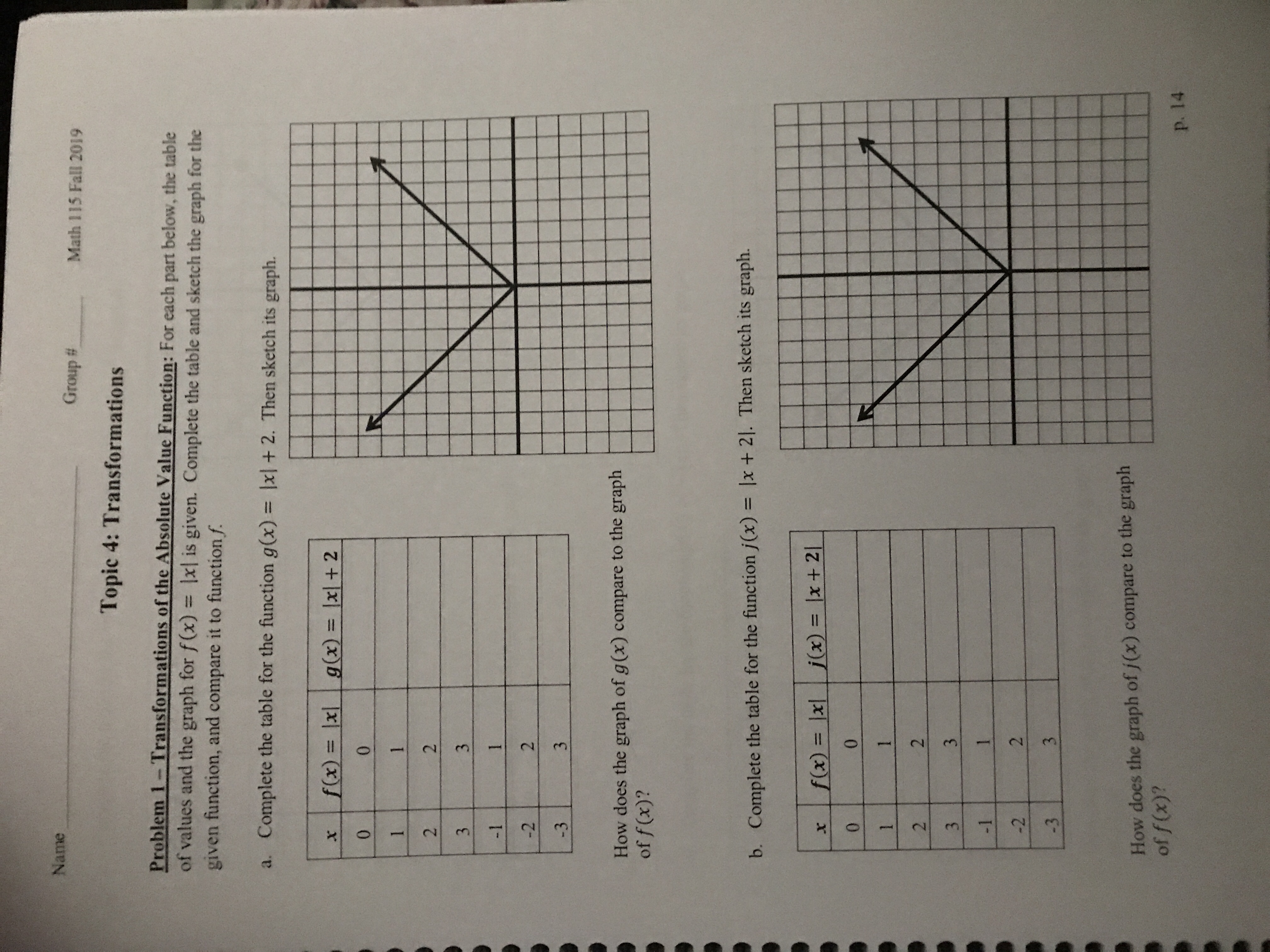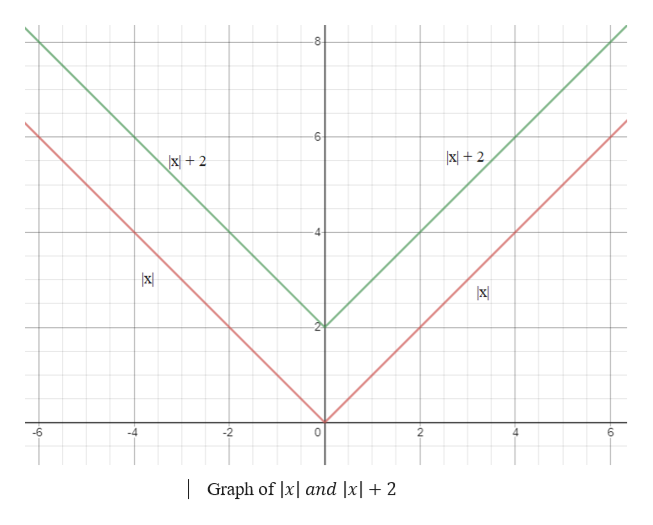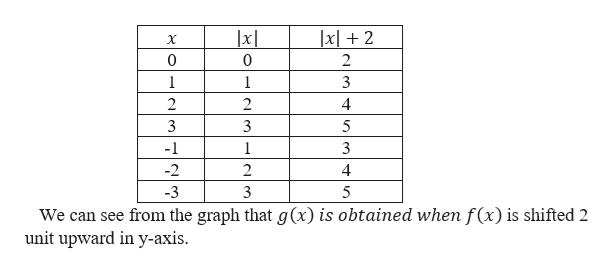# NameGroup #Math 115 Fall 2019Topic 4: TransformationsProblem 1-Transformations of the Absolute Value Function: For each part below, the tableof values and the graph for f(x) = lxl is given. Complete the table and sketch the graph for thegiven function, and compare it to function f.a. Complete the table for the function g(x) = lxl +2. Then sketch its graph.l |f(x)= lxg(x)= lxl + 2X00112331-1-223-3How does the graph of g (x) compare to the graphof f(x)?b. Complete the table for the function j(x) = lx + 21. Then sketch its graph.j(x) = |x + 21f(x) = |xx00112331-12-23-3How does the graph of /(x) compare to the graphof f (x)?p. 142/N2

Questionhelp_outlineImage TranscriptioncloseName Group # Math 115 Fall 2019 Topic 4: Transformations Problem 1-Transformations of the Absolute Value Function: For each part below, the table of values and the graph for f(x) = lxl is given. Complete the table and sketch the graph for the given function, and compare it to function f. a. Complete the table for the function g(x) = lxl +2. Then sketch its graph. l | f(x)= lx g(x)= lxl + 2 X 0 0 1 1 2 3 3 1 -1 -2 2 3 -3 How does the graph of g (x) compare to the graph of f(x)? b. Complete the table for the function j(x) = lx + 21. Then sketch its graph. j(x) = |x + 21 f(x) = |x x 0 0 1 1 2 3 3 1 -1 2 -2 3 -3 How does the graph of /(x) compare to the graph of f (x)? p. 14 2/ N 2 fullscreen
check_circle

Step 1

Graph of part ahelp_outlineImage Transcriptionclose8 |x + 2 X2 4 x -6 -4 -2 0 2 6 |Graph of lx and |x| + 2 fullscreen
Step 2

Completing the table with the help of the graph given in the step1.help_outlineImage Transcriptionclosexl2 X 0 1 1 3 2 2 4 3 3 5 -1 1 -2 2 4 -3 3 5 We can see from the graph that g(x) is obtained when f(x) is shifted 2 unit upward in y-axis fullscreen
Step 3

Graph of pa...

### Want to see the full answer?

See Solution

#### Want to see this answer and more?

Solutions are written by subject experts who are available 24/7. Questions are typically answered within 1 hour.*

See Solution
*Response times may vary by subject and question.
Tagged in

### Functions